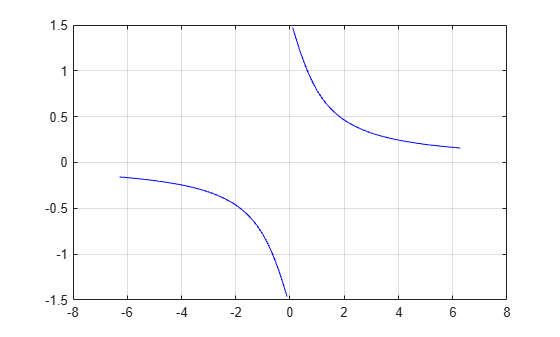Main Content

# acot

Inverse cotangent in radians

## Syntax

``Y = acot(X)``

## Description

example

````Y = acot(X)` returns the Inverse Cotangent (cot-1) of the elements of `X` in radians. The function accepts both real and complex inputs. For real values of `X`, `acot(X)` returns values in the interval [-π/2, π/2].For complex values of `X`, `acot(X)` returns complex values. ```

## Examples

collapse all

Find the inverse cotangent of a value.

`acot(2.6)`
```ans = 0.3672 ```

Find the inverse cotangent of the elements of vector `x`. The `acot` function acts on `x` element-wise.

```x = [0.5i 1+3i -2.2+i]; Y = acot(x)```
```Y = 1×3 complex 1.5708 - 0.5493i 0.1093 - 0.3059i -0.3689 - 0.1506i ```

Plot the inverse cotangent function over the intervals $-2\pi \le x<0$ and $0.

```x1 = -2*pi:pi/30:-0.1; x2 = 0.1:pi/30:2*pi; plot(x1,acot(x1),'b') hold on plot(x2,acot(x2),'b') grid on```## Input Arguments

collapse all

Cotangent of angle, specified as a scalar, vector, matrix, or multidimensional array. The `acot` operation is element-wise when `X` is nonscalar.

Data Types: `single` | `double`
Complex Number Support: Yes

## More About

collapse all

### Inverse Cotangent

The inverse cotangent is defined as

`${\mathrm{cot}}^{-1}\left(z\right)={\mathrm{tan}}^{-1}\left(\frac{1}{z}\right).$`

## See Also

Introduced before R2006a

Download ebook